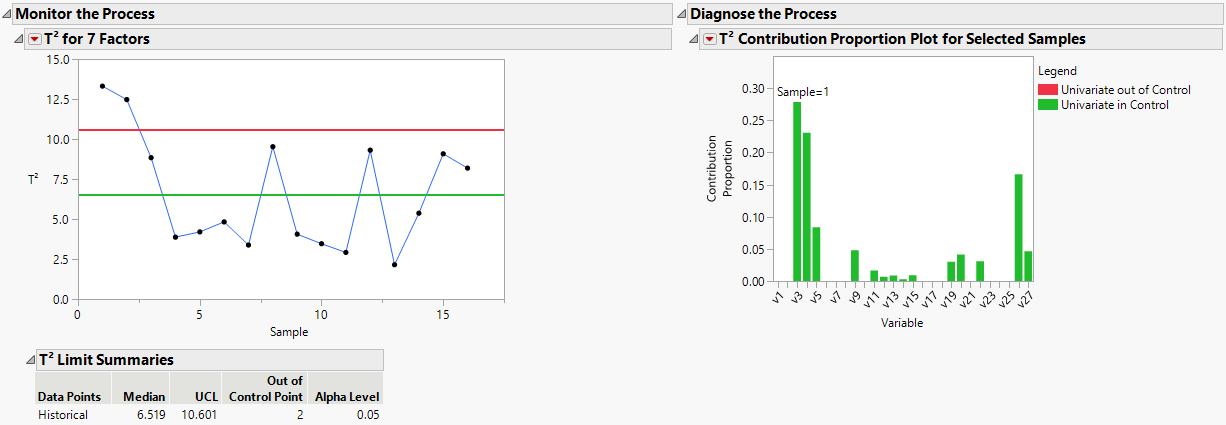Publication date: 07/30/2020

## Example of an MDMVCC with a PLS Model

This example demonstrates the use of a PLS model for monitoring a multivariate process.

1. Select Help > Sample Data Library and open Baltic.jmp.

2. Run the Partial Least Squares table script.

3. Click the NIPALS Fit with 7 Factors red triangle and select Save Columns > Save Score Formula.

4. Select Analyze > Quality and Process > Model Driven Multivariate Control Chart.

5. Select the X Score 1 Formula through X Score 7 Formula and click Process.

6. Click OK.

7. Hover over the first sample data point and click on the contribution proportion plot to open a larger version of the plot.

Figure 11.9 T2 Chart and Sample 1 Contribution Proportion PlotNotice that the contributions plot is in terms of the PLS model input variables.